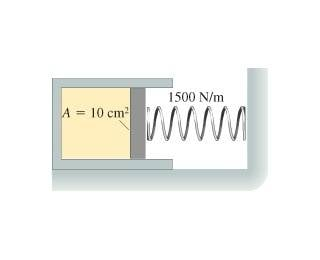# Is this correct? - Gas Law Question

Is this correct? -- Gas Law Question

## Homework StatementThe cylinder in the figure has a moveable piston attached to a spring. The cylinder's cross sectional area is 10 cm^2 and it contains .005 mol of gas. The spring constant is 1500 N/m. At 16 degrees Celsius, the spring is neither stretched nor compressed. How far is the spring compressed when the gas temperature is raised to 160 degrees Celsius?

## The Attempt at a Solution

P1/T1 = P2/T2

16 degrees C = 289 K 160 degrees C = 433 K

10cm^2 * (1m/100cm)^2 = .001 m^2

Since the spring is not exerting any force on the piston initially, the pressure inside the cylinder is equal to the air pressure (1 atm = 101300 Pa)

(101300 Pa)/(289K) = P2/(433 K)

P2=151775 Pa and P2= (1500N/m)x/(.001 m^2) + (101300 Pa)

x=.034 m = 3.4 cm

alphysicist
Homework Helper
Hi bcjochim07,

## Homework Statement

View attachment 13865
The cylinder in the figure has a moveable piston attached to a spring. The cylinder's cross sectional area is 10 cm^2 and it contains .005 mol of gas. The spring constant is 1500 N/m. At 16 degrees Celsius, the spring is neither stretched nor compressed. How far is the spring compressed when the gas temperature is raised to 160 degrees Celsius?

## The Attempt at a Solution

P1/T1 = P2/T2

This expression applies for cases when the volume is constant; I think you'll need to use the form with the volumes in it.

Oh yeah, how could I forget?

So the initial volume can be found:
PV=nRT (101,300 Pa)(V1)=(.005mol)(8.31)(289 K)
V1= .0001185 m^3

P1V1/T1 = P2V2/T2 P2= 101300 Pa + (1500N/m)x/.001 m^2 and
V2= V1 + Ax where A is the area of the end of the cylinder
V2= .0001185m^3 + (.001m^2)x

(101300 Pa)(.000185 m^3)/(289K) = (101300 Pa + 1500x/.001 m^2)(.0001185 + .001x)(1/433K)

.0648 = (101300 + 1500000x)(.0001185 +.001x)(1/433)
.0648 = -.0368 + .644 x + 3.46x^2

[-.644 +/- sqrt (.92)]/6.92

x= (-.644 + 1.03)/6.92 = .0455 m = 4.55 cm

Last edited:
Is this correct?

alphysicist
Homework Helper
I think you have a few errors here.

Oh yeah, how could I forget?

So the initial volume can be found:
PV=nRT (101,300 Pa)(V1)=(.005mol)(8.31)(289 K)
V1= .0001185 m^3

P1V1/T1 = P2V2/T2 P2= 101300 Pa + (1500N/m)x/.001 m^2 and
V2= V1 + Ax where A is the area of the end of the cylinder
V2= .0001185m^3 + (.001m^2)x

(101300 Pa)(.000185 m^3)/(289K) = (101300 Pa + 1500x/.001 m^2)(.0001185 + .001x)(1/433K)

On the left hand side you have the wrong volume (you're missing a one) that gives the wrong answer on the left side of the next line.

.0648 = (101300 + 1500000x)(.0001185 +.001x)(1/433)
.0648 = -.0368 + .644 x + 3.46x^2

I think you also made a calculation error in going between these two lines on the right hand side. The right hand side of (101300 + 1500000x)(.0001185 +.001x)(1/433) has all positive numbers, but you got a negative number on the next line.

Ok, let's see if I can get it right

(101300Pa)(.0001185 m^3)/(289K) = (101300 Pa + 1500x/.001 m^2)(.0001185 m^3 + .001x)(1/433K)

.0415 = (101300 + 1500000x)(.0001185 + .001x)(1/433)
.0415 = (12.00 + 101.3x + 177.75x + 1500x^2)(1/433)
.0415 = 3.46x^2 + .234x + .4105x + .0277
0 = 3.46x^2 + .644x - .0138
[-.644 +/- sqrt (.606)]/6.92
x= .0194 m = 1.94 cm

Is that right?

alphysicist
Homework Helper
That looks right to me.